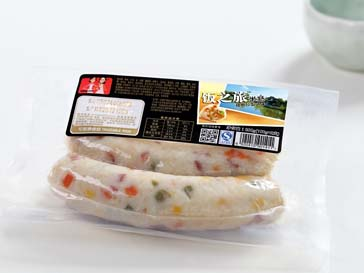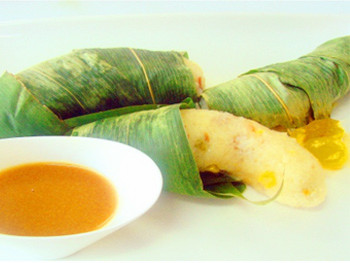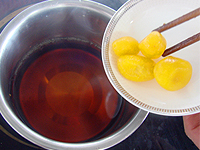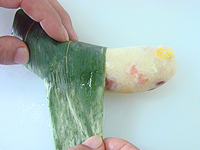﻿ 扬州炒饭 - 消费食品 - 姐妹厨房 享受安心的味道 ﻿
• 鸡柳类
• 香嫩鸡柳
• 川香鸡柳
• 金三角鸡柳
• 汉堡类
• 汉堡
• 老虎饼
• 牛肉汉堡
• 牛肉饼
• 乡美牛肉饼
• 乡美汉堡
• 五香鸡肉饼
• 超厚鸡排
• 猪肉贝帝堡（原味）
• 脆香猪排
• 甜块类
• 芒果慕斯
• 奶油玉米甜心
• 丸子类
• 御膳丸
• 四喜御膳丸
• 月亮烧
• 包心丸子
• 红烧丸子
• 原肉类
• 鸡腿汉堡
• 香鸡排
• 龙田扬
• 翅类
• 吮指炸翅根
• 吮指炸翅中
• 夹心类
• 黄金翡翠鸡派
• 台畜类
• 三明治切片
• 德式香肠
• 培根
• 香薰鸡肉片
• 台湾脆骨肠
• 土鸡类
• 香醉土鸡翅
• 香葱土鸡腿
• 香醇白斩土鸡
• 原汁土鸡汤
•• 冷冻鸡肉
• 急鲜封鸡胸肉
• 急鲜封琵琶腿
• 急鲜封翅中
• 急鲜封翅根
• 急鲜封滑嫩鸡胸肉
• 急鲜封滑嫩琵琶腿
• 飞雪土鸡
• 飞雪土鸡散养鸡
• 飞雪土鸡鸡汤
• 飞雪土鸡翅根
• 飞雪土鸡翅中尖
• 飞雪土鸡琵琶腿
• 飞雪土鸡鸡柳
• 飞雪土鸡大胸
• 鸡肉肠
• 台式鸡肉肠
• 鸡肉烤肠
• 鸡肉蒜肠
• 鸡肉红肠
• 德式鸡肉肠
• 维也纳鸡肉肠
• 台湾风味脆骨肠
• 鸡肉火腿
• 法式鸡肉火腿
• 意式鸡肉火腿
• 脆骨赢养火腿
• 鸡肝赢养火腿
• 台畜猪肉系列
• 牧风原肉火腿
• 熏腿原肉火腿
• 古早味切片火腿
• 三明治切片火腿
• Q弹火腿
• 德式香肠
• 台湾米肠
• 扬州炒饭
• 台式油饭
• 海南鸡饭
• 海南鸡饭情侣套装
• 扬州炒饭情侣套装
• 台式油饭情侣套装
• 海盗肠
• 奥尔良
• 原味
• 照烧
• 泰式甜辣
• 脆骨肠
• 台式
• 蒜香
• 奥尔良
• 香辣
• 糖熏卤味
• 糖熏鸡腿
• 糖熏鸡脖
• 糖熏鸡爪
• 阿拉善沙漠节水小米
• 谷物礼盒
•• 品 名

饭之旅米肠• 净 含 量

200g• 口 味

扬州炒饭风味• 烹调方法

微波、蒸汽、水煮• 订购热线

400-810-9066•米肠在台湾已有二十年的历史，因其美味又独具特色已经成为台湾十大知名小吃之一。饱满香糯十足的米粒，添加风味配料或肉馅，灌制成为米肠。 “bwin中文下载地址厨房”特别聘请五星酒店主厨所研发的“饭之旅米肠”，精选各国顶级食材，推出国人喜爱之各种口味，不出国也能尝遍全世界之米食。并且独有的安心码，真正实现了食品安全的可追溯性，全程透明，好吃又安心！并具有方便快捷、口味丰富、营养健康等特点。红烧香肠米肠、粽叶、生抽、金桔、冰糖、红糖将生抽、金桔、冰糖一起熬制成醮汁；用粽叶将米肠裹好后入蒸笼蒸约3～5分钟，待出清香味时即可，取出装盘。• 糯米
• 大米
• 鸡肉
• 猪肠衣
• 香菇
• 红葱
• 酱油
• 白砂糖
• 虾皮
• 芝麻香油
• 玉米淀粉
• 食用盐
• 食用香精
• 食品添加剂